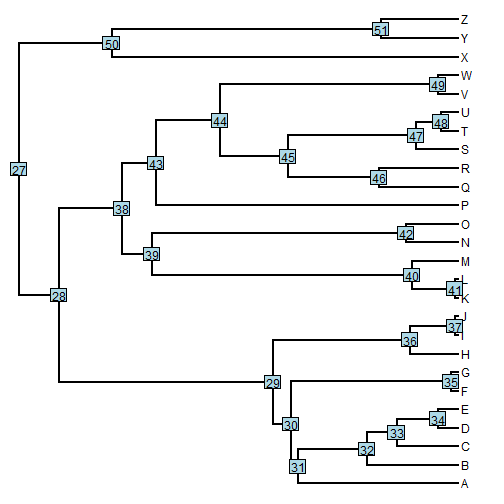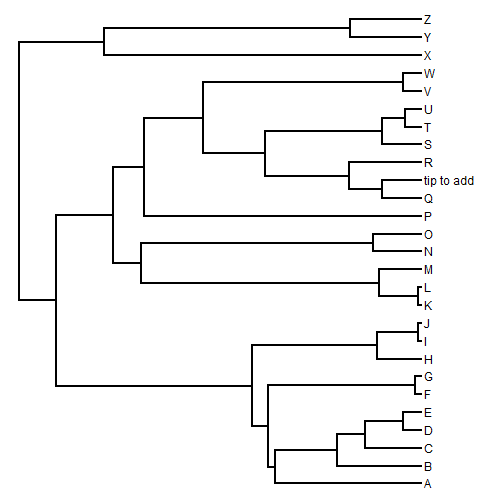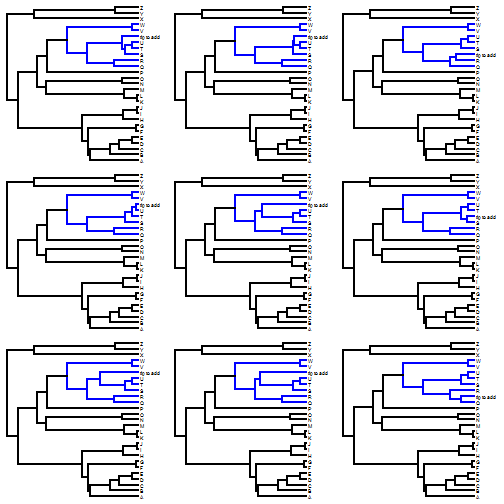## Thursday, October 5, 2017

There is a function that does this in phytools called `add.genus.to.species`; however, this is hardwired to automatically detect the genus of the tip to be added and the corresponding clade to which it should be attached. Consequently, I thought it would be easier to demonstrate how this can be done using `splitTree`, `add.random`, and `paste.tree`.

Here's an example.

First, our tree.

``````library(phytools)
plotTree(tree)
nodelabels()
``````Imagine we want to bind our tip randomly from the clade descended from the node number 45.

(Remember that we can select our node interactively using the function `getnode`.)

``````node<-45
tt<-splitTree(tree,split=list(node=node,
bp=tree\$edge.length[which(tree\$edge[,2]==node)]))
new.tree<-paste.tree(tt[],tt[])
plotTree(new.tree)
``````Let's repeat this a bunch of times:

``````foo<-function(tree,node){
tt<-splitTree(tree,split=list(node=node,
bp=tree\$edge.length[which(tree\$edge[,2]==node)]))
paste.tree(tt[],tt[])
}
new.trees<-replicate(9,foo(tree,node),simplify=FALSE)
class(new.trees)<-"multiPhylo"
``````

We can visualize the result (keeping in mind that the CA of our clade is now node 46, not 45, when we color the clade).

``````par(mfrow=c(3,3))
nulo<-sapply(new.trees,function(x) plot(paintSubTree(x,45,"2"),
colors=setNames(c("black","blue"),1:2),fsize=0.6))
``````That's it.

The tree for this example was simulated as follows:

``````tree<-pbtree(n=26,tip.label=LETTERS)
``````